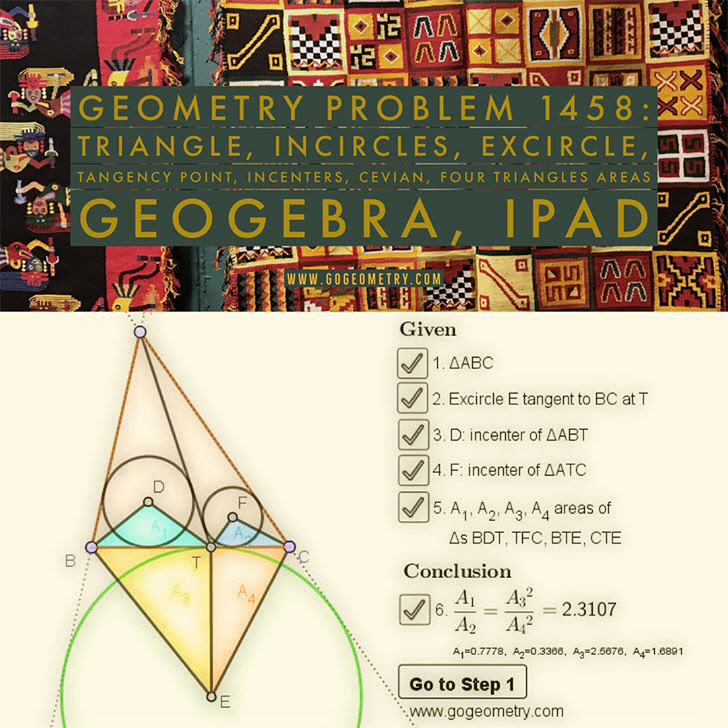## Saturday, March 7, 2020

### Geometry Problem 1458: Triangle, Incircles, Excircle, Area, Step-by-step Illustration

Interactive step-by-step animation using GeoGebra. Post your solution in the comment box below.
Level: Mathematics Education, High School, Honors Geometry, College.

Details: Click on the figure below.#### 1 comment:

1.It is easy to see that A3/A4=(s-c)/(s-b) ....(1)
Let r1,s1,S1 and r2,s2,S2 are in-radius,semi perimeter and area of triangle ABT and ACT respectively.
We have s1=s2=(s+AT)/2, s is semi perimeter of Tr.ABC
r1/r2=(S1/s1)/(S2/s2)=S1/S2=(s-c)/(s-b)
A1/A2=(r1/r2).((s-c)/(s-b))=(s-c)^2/(s-b)^2
Using eq 1, we have A1/A2=A3^2/A4^2